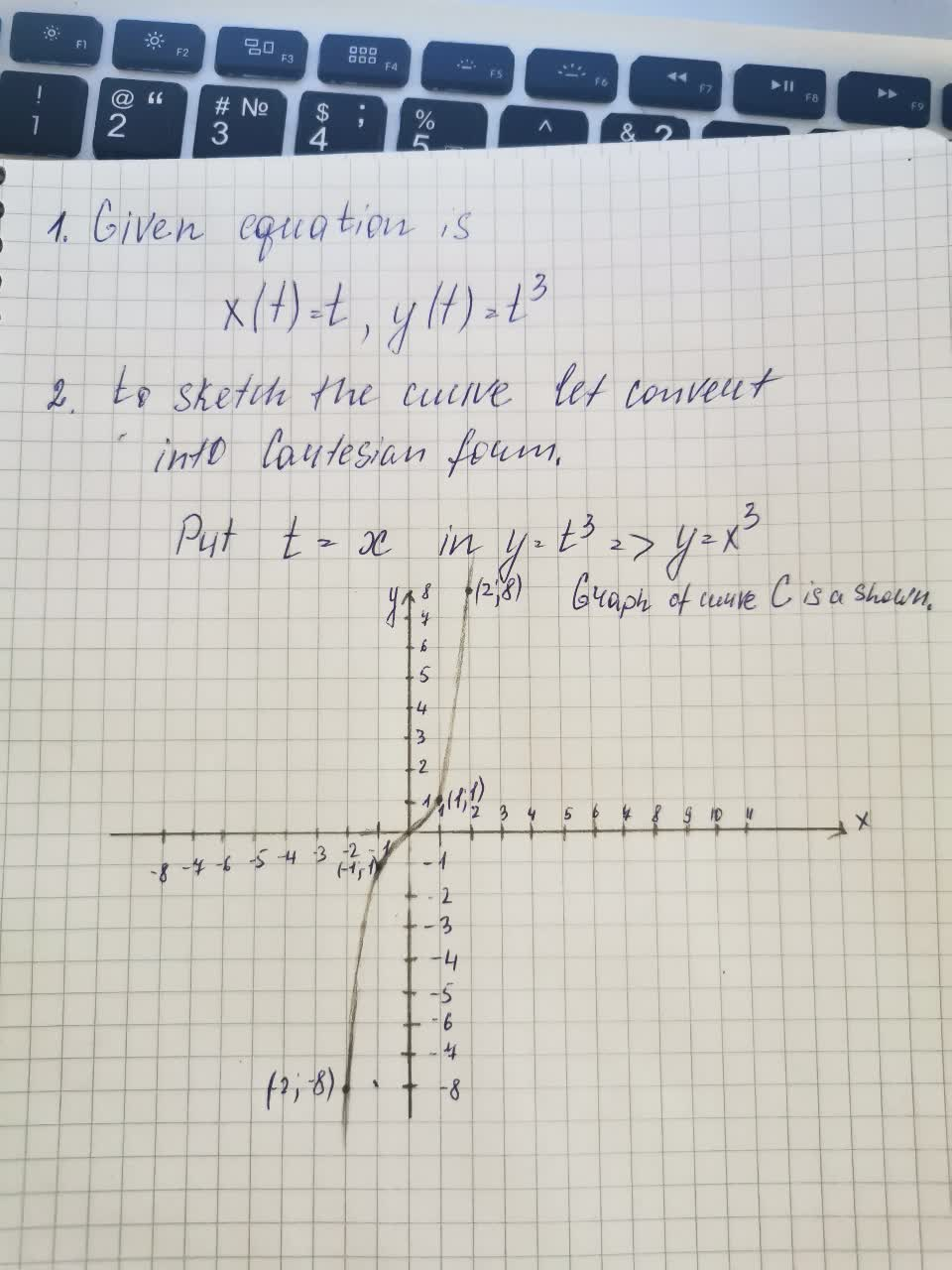# Let C be the curve described by the parametric equations x(t)= t, y(t) =t^3 a) sAlbarellak 2021-08-16 Answered
Let C be the curve described by the parametric equations x(t)= t, y(t) =$$\displaystyle{t}^{{3}}$$
a) sketch a graph of C
b) find the vector-valued function f(t) associated with this parameterization of the curve C.

• Questions are typically answered in as fast as 30 minutes

### Plainmath recommends

• Get a detailed answer even on the hardest topics.
• Ask an expert for a step-by-step guidance to learn to do it yourself.Faiza Fuller

The solution is written below